Guru

# If a, b, c, d are in continued proportion, prove that: (i) (a3+b3+c3) /(b3+c3+d3) =a/d (ii) (a2 – b2) (c2 – d2) = (b2 – c2)2 (iii) (a + d) (b + c) – (a + c) (b + d) = (b – c)2 (iv) a: d = triplicate ratio of (a – b): (b – c) (v) [ (a-b) /c+(a-c) /b]2 – [(d-b) /c +(d-c) /b)] 2 =(a-d) 2(1/c2-1/b2)

• 0

This is an important ques from the Book ML Aggarwal class 10th,, chapter – 7, ratio and proportion.

It is given that a, b, c, d are in continued proportion and we have to prove the equalities based on the fact given in the question

Question 22, 7.2

Share

1. Solution:

It is given that

a, b, c, d are in continued proportion

Here we get

a/b = b/c = c/d = k

c = dk, b = ck = dk . k = dk2

a = bk = dk2 . k = dk3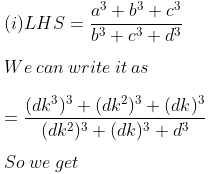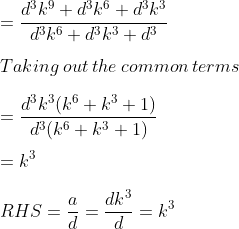Therefore, LHS = RHS.

(ii) LHS = (a2 – b2) (c2 – d2)

We can write it as

= [(dk3)2 – (dk2)2] [(dk)2 – d2]

By further calculation

= (d2k6 – d2k4) (d2k2 – d2)

Taking out the common terms

= d2k4 (k2 – 1) d2 (k2 – 1)

= d4k4 (k2 – 1)2

RHS = (b2 – c2)2

We can write it as

= [(dk2)2 – (dk)2]2

By further calculation

= [d2k4 – d2k2]2

Taking out the common terms

= [d2k2 (k2 – 1)]2

= d4 k4 (k2 – 1)2

Therefore, LHS = RHS.

(iii) LHS = (a + d) (b + c) – (a + c) (b + d)

We can write it as

= (dk3 + d) (dk2 + dk) – (dk3 + dk) (dk2 + d)

Taking out the common terms

= d (k3 + 1) dk (k + 1) – dk (k2 + 1) d (k2 + 1)

By further simplification

= d2k (k + 1) (k3 + 1) – d2k (k2 + 1) (k2 + 1)

So we get

= d2k (k4 + k3 + k + 1 – k4 – 2k2 – 1)

= d2k (k3 – 2k2 + k)

Taking k as common

= d2k2 (k2 – 2k + 1)

= d2k2 (k – 1)2

RHS = (b – c)2

We can write it as

= (dk2 – dk)2

Taking out the common terms

= d2k2 (k – 1)2

Therefore, LHS = RHS.

(iv) a: d = triplicate ratio of (a – b): (b – c) = (a – b)3: (b – c)3

We know that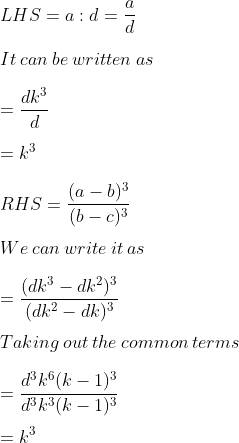Therefore, LHS = RHS.

(v)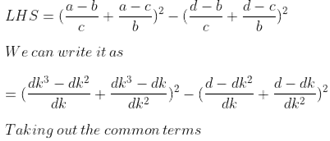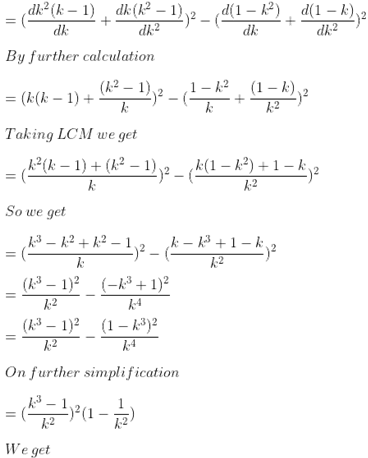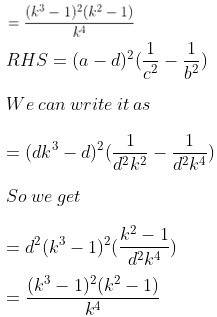Therefore, LHS = RHS.

• 0